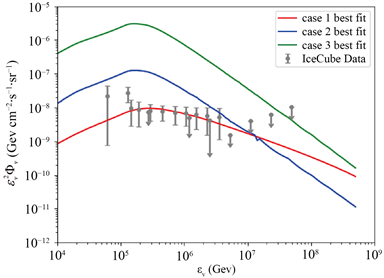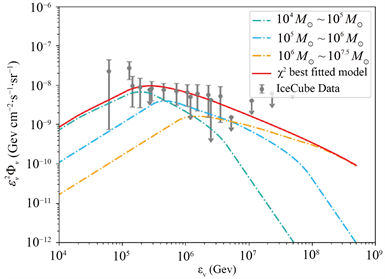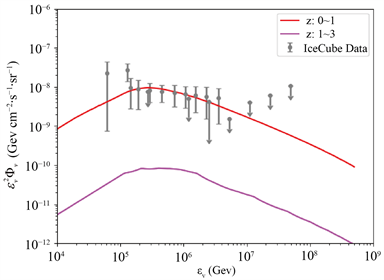#### 期刊菜单

Constraints on TDE Black Hole Mass by Diffused High Energy Neutrino Background

Abstract: Tidal Disruption Event (TDE) is one of the high-energy astrophysical events that occur around black holes. They are considered as probes of detecting black holes at the centers of quiescent galaxies, as well as sources of high-energy cosmic rays and high-energy neutrinos. In this paper, we firstly assume that the high-energy neutrino flux background observed by IceCube collaboration originated from TDEs. By constructing the TDE-produced high-energy neutrino model and fitting it to observation data, we constrain the lower limit of black hole mass and other black hole mass dependent parameters which are related to the jet fraction of TDEs and redshift-evolved black hole mass function. Our optimal model shows that when the TDE mass lies in the range of , the high-energy neutrino flux produced by jet TDEs, which dominantly contributes the peak flux of neutrino background. It also implies an anti-correlation between jet fraction of TDEs and their black hole mass, this may indicate that a large number of jet-TDEs at the low-mass end are still unveiling. In addition, our model also firstly gives the redshift-dependent evolutional index of black hole mass function, which covers the intermediate mass range that is greater than , its value is proportional to (1 + z)−2.667.

1. 引言

2. 高能中微子辐射能谱

${\epsilon }_{\nu }^{2}{\Phi }_{\nu }\left({\epsilon }_{\nu }\right)=\frac{c}{4\pi {H}_{0}}{\int }_{{M}_{\mathrm{min}}}^{{M}_{\mathrm{max}}}\text{d}M{\int }_{0}^{{z}_{\mathrm{max}}}\frac{\stackrel{˙}{\rho }\left({\epsilon }_{\nu },z,M\right)}{\sqrt{{\Omega }_{M}{\left(1+z\right)}^{3}+{\Omega }_{\Lambda }}}\text{d}z$ (1)

$\stackrel{˙}{\rho }\left({\epsilon }_{\nu },z,M\right)={\epsilon }_{\nu }^{2}{\varphi }_{\nu }\left({\epsilon }_{\nu },z,M\right)×{f}_{j}\left(M\right)×{\stackrel{˙}{N}}_{\text{TDE}}\left(M\right)×\phi \left(z,M\right)$ (2)

${f}_{j}\left(M\right)$ 代表喷流TDE的比例函数。考虑到在观测上，只有喷流张角指向地球视线方向时，才能喷流TDE才能被观测到。所以，根据文献  喷流TDE比例系数可表示为喷流张角沿视线方向的指向因子 $2{\Gamma }^{-2}$ 与归一系数 ${\eta }_{0}$ 的乘积， ${\eta }_{0}$ 通常取值为0.1 。然而，目前观测认证的具有喷流结构的TDE样本数目有限，对于这类群体的统计性质以及触发产生喷流的关键因素我们还知之甚少。此外，相较于前人的研究，我们的研究还发现，fj与黑洞质量有紧密的相关关系。因此在本文中，我们假设fj与黑洞质量呈指数变化关系，同时设 ${\eta }_{0}$${\alpha }_{f}$ 为自由变量用以拟合模型。表达式如下：

${f}_{j}\left(M\right)=\frac{{\eta }_{0}}{2{\Gamma }^{2}}{\left(\frac{M}{{10}^{6}{M}_{\odot }}\right)}^{{\alpha }_{f}}$ (3)

${\stackrel{˙}{N}}_{\text{TDE}}$ 是发生TDE的概率。TDE可以看成黑洞引力场捕获恒星的过程，理论上依据loss-cone动力学估算TDE发生概率  ，大小为 ${10}^{\text{-}4}~{10}^{-5}{\text{yr}}^{-1}\cdot {\text{gal}}^{-1}$。然而，由于M-σ关系为观测的经验关系，一般适用黑洞质量区间下限设为 ${10}^{5}{M}_{\odot }$。在考虑质量低至 ${10}^{4}{M}_{\odot }$ 量级的黑洞以及核星团对TDE 发生概率的增强作用后 ：

${\stackrel{˙}{N}}_{\text{TDE}}\left(M\right)=4×{10}^{-5}{\left(\frac{M}{{M}_{\odot }}\right)}^{-0.14}{\text{yr}}^{-1}\cdot {\text{gal}}^{-1}$ (4)

$\varphi \left(\text{z},M\right)$ 代表黑洞的质量函数。黑洞的质量函数用以描述不同红移处、不同黑洞质量间隔内黑洞数目的分布，因而直接反映了黑洞与星系共同演化的历史。然而，受限于观测灵敏度，目前黑洞质量函数多为超大质量黑洞的质量函数，其质量区间为 ${10}^{5}{M}_{\odot }~{10}^{9}{M}_{\odot }$，对中等质量黑洞的质量函数知之甚少。对于小于质量端黑洞的分布情况认知尚浅。基于前人的工作，文章  首次推导出本地宇宙( $z\le 0.1$ )中覆盖部分中等质量范围的黑洞质量函数。其函数如下：

${\mathrm{log}}_{10}\left(\phi \left(M\right)\right)={c}_{1}+{c}_{2}{\mathrm{log}}_{10}\left(M/{M}_{\odot }\right)+{c}_{3}\mathrm{exp}\left({\mathrm{log}}_{10}\left(M/{M}_{\odot }\right)\right)$ (5)

$\phi \left(z,M\right)=\phi \left(M\right)×{\left(1+z\right)}^{-{\alpha }_{z}}$ (6)

3. HESE观测数据和模型拟合

${\chi }^{2}={\underset{i}{\overset{N}{\sum }}\left(\frac{{\Phi }_{\nu ,i}^{mod}-{\Phi }_{\nu ,i}^{obs}}{{\epsilon }_{i}^{obs}}\right)}^{2}$

4. 结论

4.1. 三种模型Table 1. Best-fitted parameters of three casesFigure 1. High-energy neutrino spectrum of three cases

4.2. 最优模型Figure 2. The best-fitted model component analysis

${f}_{j}\left(M\right)=8.505×{10}^{-3}{\left(\frac{M}{{10}^{6}{M}_{\odot }}\right)}^{-0.374}$ (7)

$M={10}^{6}{M}_{\odot }$ 时，计算可得 ${f}_{j}=8.505×{10}^{-3}$，满足文献  中基于观测分析的限制，即 $3×{10}^{-3}<{f}_{j}<1$。公式(7)中的指数等于−0.374，这意味着在TDEs中，随着M的增加，fj比例减少。这种反相关关系或许与不同质量区间的流量贡献比例直接相关。然而，由于当前缺乏中等质量黑洞TDE的观测样本，它仍然需要依赖今后的天文巡天观测数据，予以参数空间的进一步限制及验证。

5. 总结Figure 3. Comparison of high-energy neutrino flux between higher and lower redshift

NOTES

1https://tde.space/。

  Rees, M.J. (1988) Tidal Disruption of Stars by Black Holes of 106-108 Solar Masses in Nearby Galaxies. Nature, 333, 523-528. https://doi.org/10.1038/333523a0  Dai, L., McKinney, J.C., Roth, N., et al. (2018) A Unified Model for Tidal Disruption Events. The Astrophysical Journal Letters, 859, L20. https://doi.org/10.3847/2041-8213/aab429  Auchettl, K., Guillochon, J. and Ramirez-Ruiz, E. (2017) New Physical Insights about Tidal Disruption Events from a Comprehensive Observational Inventory at X-Ray Wavelengths. The Astrophysical Journal Letters, 838, 149. https://doi.org/10.3847/1538-4357/aa633b  van Velzen, S., Gezari, S., Hammerstein, E., et al. (2020) Seven-teen Tidal Disruption Events from the First Half of ZTF Survey Observations: Entering a New Era of Population Studies. The Astrophysical Journal Letters, 908, 4V. https://doi.org/10.3847/1538-4357/abc258  Komossa, S. (2015) Tidal Disruption of Stars by Supermassive Black Holes: Status of Observations. Journal of High Energy Astrophysics, 7, 148-157. https://doi.org/10.1016/j.jheap.2015.04.006  Piran, T. and Krolik, J. (2012) Time Scales in Tidal Disruption Events. European Physical Journal Web of Conferences, 39, Article No. 02006. https://doi.org/10.1051/epjconf/20123902006  Burrows, D.N., Kennea, J.A., Ghisellini, G., et al. (2011) Re-lativistic Jet Activity from the Tidal Disruption of a Star by a Massive Black Hole. Nature, 476, 421-424. https://doi.org/10.1038/nature10374  Cenko, S.B., Krimm, H.A., Horesh, A., et al. (2012) Swift J2058.4+0516: Discovery of a Possible Second Relativistic Tidal Disruption Flare? The Astrophysical Journal Letters, 753, Article No. 77. https://doi.org/10.1088/0004-637X/753/1/77  Tchekhovskoy, A., Metzger, B.D., Giannios, D., et al. (2014) Swift J1644+57 Gone MAD: The Case for Dynamically Important Magnetic Flux Threading the Black Hole in a Jetted Tidal Disruption Event. Monthly Notices of the Royal Astronomical Society, 437, 2744-2760. https://doi.org/10.1093/mnras/stt2085  Curd, B. and Narayan, R. (2019) GRRMHD Simulations of Tidal Disruption Event Accretion Discs around Supermassive Black Holes: Jet Formation, Spectra, and Detectability. Monthly Notices of the Royal Astronomical Society, 483, 565-592. https://doi.org/10.1093/mnras/sty3134  Biehl, D., Boncioli, D., Lunardini, C. and Winter, W. (2018) Tidally Disrupted Stars as a Possible Origin of Both Cosmic rays and Neutrinos at the Highest Energies. Scientific Reports, 8, Article No. 10828. https://doi.org/10.1038/s41598-018-29022-4  Lunardini, C. and Winter, W. (2017) High Energy Neutrinos from the Tidal Disruption of Stars. Physical Review D, 95, Article ID: 123001. https://doi.org/10.1103/PhysRevD.95.123001  Stein, R., van Velzen, S., Kowalski, M., et al. (2020) A High-Energy Neutrino Coincident with a Tidal Disruption Event.  Becker, J.K. (2008) High-Energy Neutrinos in the Context of Multimessenger astrophysics. Physics Reports, 458, 173-246. https://doi.org/10.1016/j.physrep.2007.10.006  IceCube Collaboration, Aartsen, M.G., Ackermann, M., et al. (2017) The IceCube Neutrino Observatory—Contributions to ICRC 2017 Part II: Properties of the Atmospheric and As-trophysical Neutrino Flux.  Wang, X.-Y., Liu, R.-Y., Dai, Z.-G., et al. (2011) Probing the Tidal Disruption Flares of Massive Black Holes with High-Energy Neutrinos. Physical Review D, 84, Article ID: 081301. https://doi.org/10.1103/PhysRevD.84.081301  Merritt, D. (2013) Loss-Cone Dynamics. Classical and Quan-tum Gravity, 30, Article ID: 244005. https://doi.org/10.1088/0264-9381/30/24/244005  Stone, N.C. and Metzger, B.D. (2016) Rates of Stellar Tidal Disruption as Probes of the Supermassive Black Hole Mass Function. Monthly Notices of the Royal Astronomical Society, 455, 859-883. https://doi.org/10.1093/mnras/stv2281  Pfister, H., Volonteri, M., Lixin Dai, J. and Colpi, M. (2020) En-hancement of the Tidal Disruption Event Rate in Galaxies with a Nuclear Star Cluster: From Dwarfs to Ellipticals. Monthly Notices of the Royal Astronomical Society, 497, 2276-2285. https://doi.org/10.1093/mnras/staa1962  Wevers, T., Stone, N.C., van Velzen, S., et al. (2019) Black Hole Masses of Tidal Disruption Event Host Galaxies II. Monthly Notices of the Royal Astronomical Society, 487, 4136-4152. https://doi.org/10.1093/mnras/stz1602  Gallo, E. and Sesana, A. (2019) Exploring the Local Black Hole Mass Function below 106 Solar Masses. The Astrophysical Journal Letters, 883, L18A. https://doi.org/10.3847/2041-8213/ab40c6  Shankar, F., Weinberg, D.H. and Miralda-Escudé, J. (2009) Self-Consistent Models of the AGN and Black Hole Populations: Duty Cycles, Accretion Rates, and the Mean Radiative Efficiency. The Astrophysical Journal Letters, 690, 20-41. https://doi.org/10.1088/0004-637X/690/1/20  Pandey, M., Majumdar, D. and Halder, A. (2019) IceCube PeV Neutrino Events from the Decay of Superheavy Dark Matter: An Analysis.  De Colle, F. and Lu, W. (2019) Jets from Tidal Disruption Events. New Astronomy Reviews, 89, Article ID: 101538. https://doi.org/10.1016/j.newar.2020.101538